# Solution Example II

Let us define the z-axis equal to to the direction of the angular velocity vector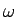. The variables in the coordinate system K and those in the co-rotational system K' are related by the rotation matrix as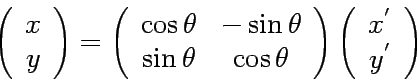(10)

where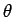is an angle between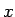-axis in K and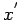-axis in K'. Note that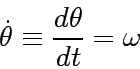(11)

and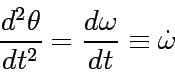(12)

Then the time differential operation applied in the equation (10) gives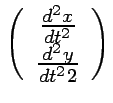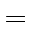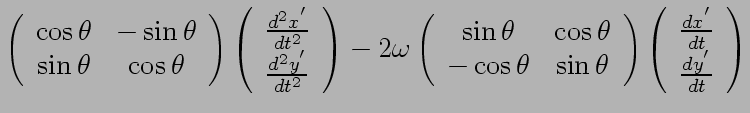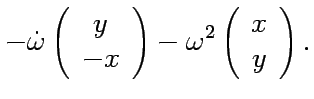(13)

Here we occasionally used the relation (10) whenever applicable. Multiplication of the inversed rotation matrix from left-side to the equation above then leads to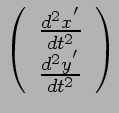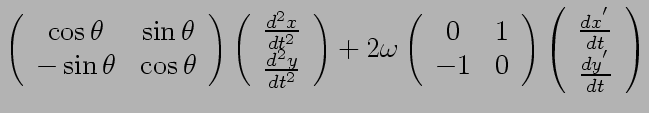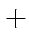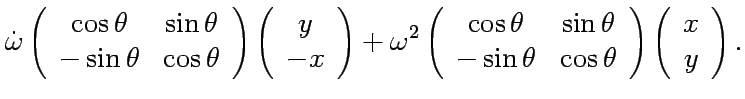Using the Newtonian equation of motion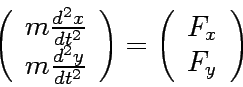(14)

where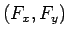is the external force of the x and y component, respectively, and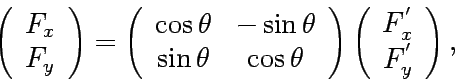(15)

we finally obtain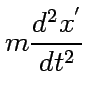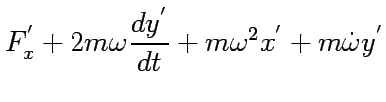(16)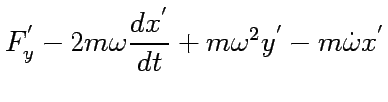(17)

One finds the apparent force term by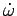.